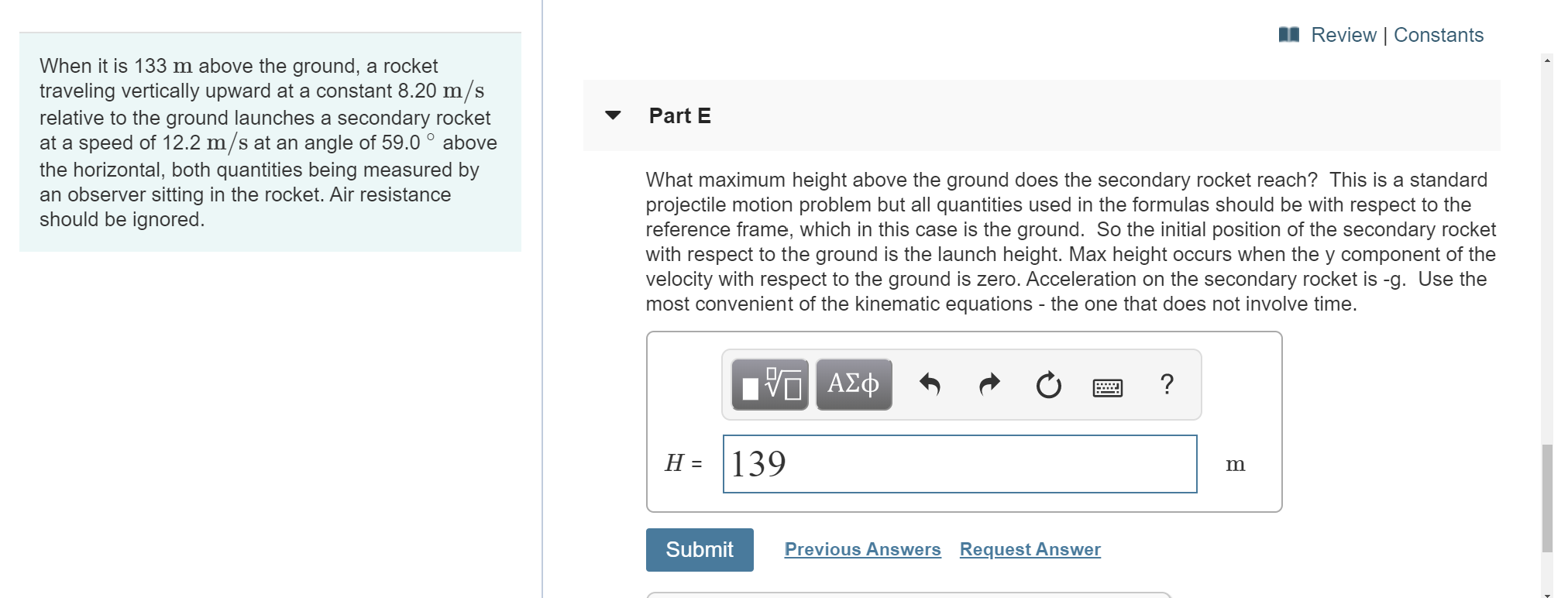# Review | Constants When it is 133 m above the ground, a rocket traveling vertically upward at a constant 8.20 m/s relative to the ground launches a secondary rocket at a speed of 12.2 m/s at an angle of 59.0° above the horizontal, both quantities being measured by an observer sitting in the rocket. Air resistance should be ignored. Part E What maximum height above the ground does the secondary rocket reach? This is a standard projectile motion problem but all quantities used in the formulas should be with respect to the reference frame, which in this case is the ground. So the initial position of the secondary rocket with respect to the ground is the launch height. Max height occurs when the y component of the velocity with respect to the ground is zero. Acceleration on the secondary rocket is -g. Use the most convenient of the kinematic equations the one that does not involve time. V ΑΣφ 139 Н- m Previous Answers Request Answer Submit

Question

I don't understand how to find the height. I had gotten 139 m as my answer but it is not correct.help_outlineImage TranscriptioncloseReview | Constants When it is 133 m above the ground, a rocket traveling vertically upward at a constant 8.20 m/s relative to the ground launches a secondary rocket at a speed of 12.2 m/s at an angle of 59.0° above the horizontal, both quantities being measured by an observer sitting in the rocket. Air resistance should be ignored. Part E What maximum height above the ground does the secondary rocket reach? This is a standard projectile motion problem but all quantities used in the formulas should be with respect to the reference frame, which in this case is the ground. So the initial position of the secondary rocket with respect to the ground is the launch height. Max height occurs when the y component of the velocity with respect to the ground is zero. Acceleration on the secondary rocket is -g. Use the most convenient of the kinematic equations the one that does not involve time. V ΑΣφ 139 Н- m Previous Answers Request Answer Submit fullscreen